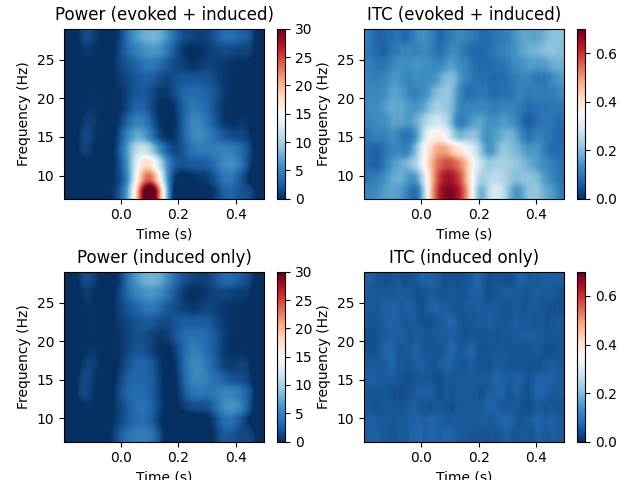# Compute power and phase lock in label of the source space¶

Compute time-frequency maps of power and phase lock in the source space. The inverse method is linear based on dSPM inverse operator.

The example also shows the difference in the time-frequency maps when they are computed with and without subtracting the evoked response from each epoch. The former results in induced activity only while the latter also includes evoked (stimulus-locked) activity.

```# Authors: Alexandre Gramfort <alexandre.gramfort@inria.fr>
#

import numpy as np
import matplotlib.pyplot as plt

import mne
from mne import io
from mne.datasets import sample

print(__doc__)
```

Set parameters

```data_path = sample.data_path()
raw_fname = data_path + '/MEG/sample/sample_audvis_raw.fif'
fname_inv = data_path + '/MEG/sample/sample_audvis-meg-oct-6-meg-inv.fif'
label_name = 'Aud-rh'
fname_label = data_path + '/MEG/sample/labels/%s.label' % label_name

tmin, tmax, event_id = -0.2, 0.5, 2

# Setup for reading the raw data
events = mne.find_events(raw, stim_channel='STI 014')

include = []

# Picks MEG channels
picks = mne.pick_types(raw.info, meg=True, eeg=False, eog=True,

epochs = mne.Epochs(raw, events, event_id, tmin, tmax, picks=picks,
baseline=(None, 0), reject=reject,

# Compute a source estimate per frequency band including and excluding the
# evoked response
freqs = np.arange(7, 30, 2)  # define frequencies of interest
n_cycles = freqs / 3.  # different number of cycle per frequency

# subtract the evoked response in order to exclude evoked activity
epochs_induced = epochs.copy().subtract_evoked()

plt.close('all')

for ii, (this_epochs, title) in enumerate(zip([epochs, epochs_induced],
['evoked + induced',
'induced only'])):
# compute the source space power and the inter-trial coherence
power, itc = source_induced_power(
this_epochs, inverse_operator, freqs, label, baseline=(-0.1, 0),
baseline_mode='percent', n_cycles=n_cycles, n_jobs=1)

power = np.mean(power, axis=0)  # average over sources
itc = np.mean(itc, axis=0)  # average over sources
times = epochs.times

##########################################################################
# View time-frequency plots
plt.subplots_adjust(0.1, 0.08, 0.96, 0.94, 0.2, 0.43)
plt.subplot(2, 2, 2 * ii + 1)
plt.imshow(20 * power,
extent=[times, times[-1], freqs, freqs[-1]],
aspect='auto', origin='lower', vmin=0., vmax=30., cmap='RdBu_r')
plt.xlabel('Time (s)')
plt.ylabel('Frequency (Hz)')
plt.title('Power (%s)' % title)
plt.colorbar()

plt.subplot(2, 2, 2 * ii + 2)
plt.imshow(itc,
extent=[times, times[-1], freqs, freqs[-1]],
aspect='auto', origin='lower', vmin=0, vmax=0.7,
cmap='RdBu_r')
plt.xlabel('Time (s)')
plt.ylabel('Frequency (Hz)')
plt.title('ITC (%s)' % title)
plt.colorbar()

plt.show()
```Out:

```Opening raw data file /home/circleci/mne_data/MNE-sample-data/MEG/sample/sample_audvis_raw.fif...
Read a total of 3 projection items:
PCA-v1 (1 x 102)  idle
PCA-v2 (1 x 102)  idle
PCA-v3 (1 x 102)  idle
Range : 25800 ... 192599 =     42.956 ...   320.670 secs
320 events found
Event IDs: [ 1  2  3  4  5 32]
Reading inverse operator decomposition from /home/circleci/mne_data/MNE-sample-data/MEG/sample/sample_audvis-meg-oct-6-meg-inv.fif...
[done]
[done]
305 x 305 full covariance (kind = 1) found.
Read a total of 4 projection items:
PCA-v1 (1 x 102) active
PCA-v2 (1 x 102) active
PCA-v3 (1 x 102) active
Average EEG reference (1 x 60) active
22494 x 22494 diagonal covariance (kind = 2) found.
22494 x 22494 diagonal covariance (kind = 6) found.
22494 x 22494 diagonal covariance (kind = 5) found.
Did not find the desired covariance matrix (kind = 3)
Computing patch statistics...
[done]
Computing patch statistics...
[done]
Read a total of 4 projection items:
PCA-v1 (1 x 102) active
PCA-v2 (1 x 102) active
PCA-v3 (1 x 102) active
Average EEG reference (1 x 60) active
Source spaces transformed to the inverse solution coordinate frame
73 matching events found
Setting baseline interval to [-0.19979521315838786, 0.0] sec
Applying baseline correction (mode: mean)
Created an SSP operator (subspace dimension = 3)
3 projection items activated
Rejecting  epoch based on EOG : ['EOG 061']
Rejecting  epoch based on EOG : ['EOG 061']
Rejecting  epoch based on EOG : ['EOG 061']
Rejecting  epoch based on EOG : ['EOG 061']
Rejecting  epoch based on EOG : ['EOG 061']
Rejecting  epoch based on MAG : ['MEG 1711']
Rejecting  epoch based on EOG : ['EOG 061']
Rejecting  epoch based on EOG : ['EOG 061']
Rejecting  epoch based on EOG : ['EOG 061']
Rejecting  epoch based on EOG : ['EOG 061']
Rejecting  epoch based on EOG : ['EOG 061']
Rejecting  epoch based on EOG : ['EOG 061']
Rejecting  epoch based on EOG : ['EOG 061']
Rejecting  epoch based on EOG : ['EOG 061']
Subtracting Evoked from Epochs
The following channels are not included in the subtraction: EOG 061
[done]
Preparing the inverse operator for use...
Scaled noise and source covariance from nave = 1 to nave = 1
Created the regularized inverter
Created an SSP operator (subspace dimension = 3)
Created the whitener using a noise covariance matrix with rank 302 (3 small eigenvalues omitted)
Computing noise-normalization factors (dSPM)...
[done]
Picked 305 channels from the data
Computing inverse...
Eigenleads need to be weighted ...
Reducing data rank 81 -> 81
Computing source power ...
Applying baseline correction (mode: percent)
Preparing the inverse operator for use...
Scaled noise and source covariance from nave = 1 to nave = 1
Created the regularized inverter
Created an SSP operator (subspace dimension = 3)
Created the whitener using a noise covariance matrix with rank 302 (3 small eigenvalues omitted)
Computing noise-normalization factors (dSPM)...
[done]
Picked 305 channels from the data
Computing inverse...
Eigenleads need to be weighted ...
Reducing data rank 81 -> 81
Computing source power ...
Applying baseline correction (mode: percent)
```

Total running time of the script: ( 0 minutes 21.797 seconds)

Estimated memory usage: 98 MB

Gallery generated by Sphinx-Gallery